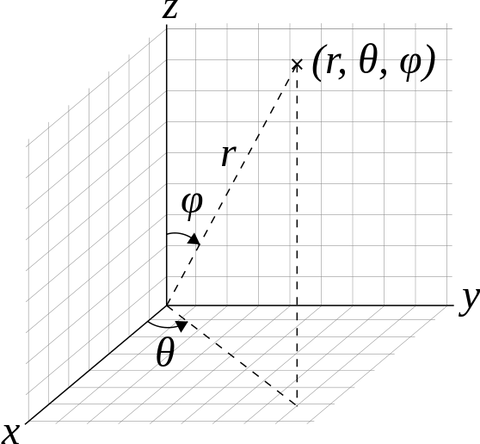# Spherical Coordinate System

### Spherical Coordinate System as commonly used in physics

Spherical coordinates (r, θ, φ) as commonly used in physics: radial distance r, polar angle θ (theta), and azimuthal angle φ (phi). The symbol ρ (rho) is often used instead of r.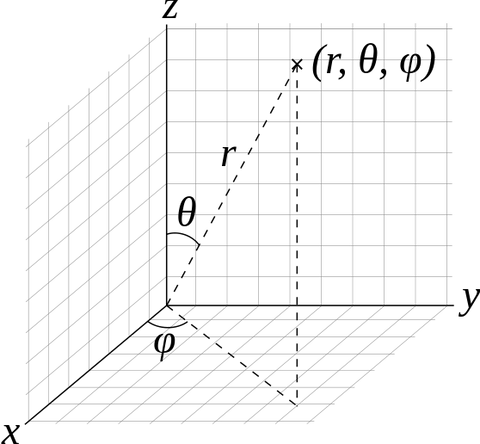### Coordinate system conversions

As the spherical coordinate system is only one of many three-dimensional coordinate systems, there exist equations for converting coordinates between the spherical coordinate system and others.

#### Cartesian coordinates

The spherical coordinates of a point in the ISO convention (radius r, inclination θ, azimuth φ) can be obtained from its Cartesian coordinates (x, y, z) by the formulae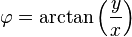Conversely, the Cartesian coordinates may be retrieved from the spherical coordinates (radius r, inclination θ, azimuth φ), where r ∈ [0, ∞), θ ∈ [0, π], φ ∈ [0, 2π), by: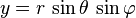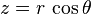### Spherical Coordinate System as commonly used in mathematics

Spherical coordinates (r, θ, φ) as often used in mathematics: radial distance r, azimuthal angle θ, and polar angle φ. The meanings of θ and φ have been swapped compared to the physics convention.